# Class 12 Maths NCERT Solutions for Chapter 11 Three Dimensional Geometry Exercise 11.2### Three Dimensional Geometry Exercise 11.2 Solutions

1. Show that the three lines with direction cosines 12/13, -3/13, -4/13; 4/13, 12/13, 3/13; 3/13, -4/13, 12/13 are mutually perpendicular.

Solution

Two lines with direction cosines, l1m1n1 and l2m2n2, are perpendicular to each other, if l1l2 + m1m2 + n1n2 = 0
(i) For the lines with direction cosines, 12/3, -3/13, -4/13 and 4/13, 12/13, 3/13, we obtain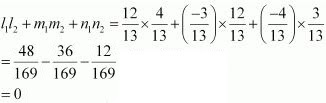Therefore, the lines are perpendicular.

(ii) For the lines with direction cosines, 4/13, 12/13, 3/13 and 3/13, -4/13, 12/13, we obtain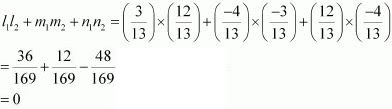Therefore, the lines are perpendicular.

(iii) For the lines with direction cosines, 3/13, -4/13, 12/13 and 12/13, -3/13, -4/13, we obtain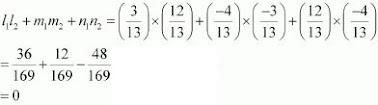Therefore, the lines are perpendicular.
Thus, all the lines are mutually perpendicular.

2. Show that the line through the points (1, −1, 2) (3, 4, −2) is perpendicular to the line through the points (0, 3, 2) and (3, 5, 6).

Solution

Let AB be the line joining the points, (1, −1, 2) and (3, 4, − 2), and CD be the line joining the points, (0, 3, 2) and (3, 5, 6).
The direction ratios, a1b1c1, of AB are (3 −1), (4 −(−1)), and (−2− 2) i.e., 2, 5, and −4.
The direction ratios, a2b2c2, of CD are (3 − 0), (5 − 3), and (6 −2) i.e., 3, 2, and 4.
AB and CD will be perpendicular to each other, if a1a2 + b1b2c1c2 = 0
a1a2 + b1b2c1c2 = 2 × 3 + 5 × 2 + (− 4) × 4
= 6 + 10 − 16
= 0
Therefore, AB and CD are perpendicular to each other.

3. Show that the line through the points (4, 7, 8) (2, 3, 4) is parallel to the line through the points (−1, −2, 1), (1, 2, 5).

Solution

Let AB be the line through the points, (4, 7, 8) and (2, 3, 4), and CD be the line through the points, (−1, −2, 1) and (1, 2, 5).
The directions ratios, a1b1c1, of AB are (2 −4), (3 −7), and (4 −8) i.e., −2, −4, and −4.
The direction ratios, a2b2c2, of CD are (1 −(−1)), (2 −(−2)), and (5 − 1) i.e., 2, 4, and 4.
AB will be parallel to CD, if  a1/a2b1/b2 , c1/c2,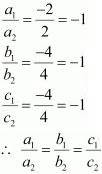Thus, AB is parallel to CD.

4. Find the equation of the line which passes through the point (1, 2, 3) and is parallel to the vector 3i ^+ 2j ^- 2k ^.

Solution

It is given that the line passes through the point A(1, 2, 3). Therefore, the position vector through A is    a⃗ = i + 2j - 3k ,  b⃗ = 3i + 2j - 2k ^
It is known that the line which passes through point A and parallel to b⃗  is given by  r⃗  = a + λb , where λ is a constant.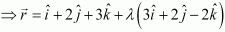This is the required equation of the line.

5. Find the equation of the line in vector and in Cartesian form that passes through the point with position vector 2i ^- j ^+ 4k ^ and is in the direction  i + 2j - k .

Solution

It is given that the line passes through the point with position vector
a⃗ = i + 2j - 3k ^  ...(1)
b⃗ = 3i + 2j - 2k ^  ...(2)
It is known that a line through a point with position vector  a and parallel to b⃗ is given by the equation,  r⃗  = a⃗ + λb⃗
⇒ r⃗ = 2i - j + 4k  + λ( i + 2j - k
This is the required equation of the line in vector form.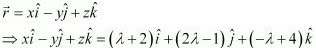Eliminating λ, we obtain the Cartesian form equation as
(x -2)/1 = (y + 1)/2 = (z- 4)/-1
This is the required equation of the given line in Cartesian form.

6. Find the Cartesian equation of the line which passes through the point (-2, 4, -5) and parallel to the line given by (x + 3)/3 = (y - 4)/5 = (z + 8)/6

Solution

It is given that the line passes through the point (-2, 4, -5) and is parallel to (x + 3)/3 = (y - 4)/5 = (z + 8)/6
The direction ratios of the line, (x + 3)/3 = (y - 4)/5 = (z + 8)/6 , are 3, 5 and 6.
The required line is parallel to (x + 3)/3 = (y - 4)/5 = (z + 8)/6
Therefore, its direction ratios are 3k, 5k, and 6k, where k ≠ 0
It is known that the equation of the line through the point ( x1y1z1) and with direction ratios, a, b, c, is given by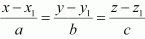Therefore the equation of the required line is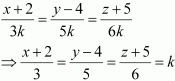7. The Cartesian equation of a line is (x - 5)/3 = (y + 4)/7 = (z - 6)/2 . Write its vector form.

Solution

The cartesian equation of the line is (x - 5)/3 = (y + 4)/7 = (z - 6)/2  ...(1)
The given line passes through the point (5, -4, 6). The position vector of this point is
a⃗ = 5i - 4j +6k
Also, the direction ratios of the given line are 3, 7, and 2.
This means that the line is in the direction of vector, b⃗ = 3i + 7j + 2k ^
It is known that the line through position vector a⃗ and in the direction of the vector b⃗  is given by the equation, r⃗ = a⃗ + λb⃗ , λ ∊ R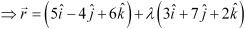This is the required equation of the given line in vector form.

8. Find the vector and the Cartesian equations of the lines that pass through the origin and (5, −2, 3).

Solution

The required line passes through the origin. Therefore, its position vector is given by,
a⃗  = 0⃗   ...(1)
The direction ratios of the line through origin and (5, -2, 3) are
(5 - 0) = 5, (-2 - 0) = -2, (3, 0) = 3
The line is parallel to the vector given by the equation b⃗ = 5i - 2j + 3k
The equation of the line in vector form through a point with position vector a⃗  and parallel to b⃗  is, r = a⃗ + λb⃗ , λ ∊ R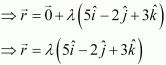The equation of the line through the point (x1y1z1) and direction ratios abc is given by,Therefore, the equation of the required line in the Cartesian form is
(x - 0)/5 = (y - 0)/-2 = (z - 0)/3
⇒ x/5 = y/-2 = z/3

9. Find the vector and the Cartesian equations of the line that passes through the points (3, −2, −5), (3, −2, 6).

Solution

Let the line passing through the points, P (3, −2, −5) and Q (3, −2, 6), be PQ.
Since PQ passes through P (3, −2, −5), its position vector is given by,
a⃗ = 3i - 2j - 5k ^
The direction ratios of PQ are given by,
(3 - 3) = 0, (-2 + 2) = 0 , (6 + 5) = 11
The equation of the vector in the direction of PQ is
b⃗ = 0.i - 0.j + 11k  = 11k ^
The equation of PQ in Cartesian form is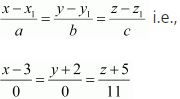10. Find the angle between the following pairs of lines:
(i) r⃗ = 2i ^- 5j + k ^+ λ (3i - 2j + 6k ^) and
r⃗ = 7i -   6k + μ (i + 2j + 2k ^
(ii) r⃗ = 3i + j ^- 2k ^ + λ (i - j ^- 2k ^) and
r⃗ = 2i - j ^- 56k ^ + μ (3i - 5j - 4k ^

Solution

(i) Let Q be the angle between the given lines.
The angle between the given pairs of lines is given by, cos Q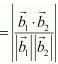The given lines are parallel to the vectors,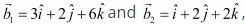respectively.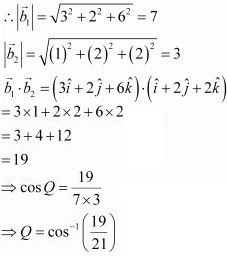(ii) The given lines are parallel to the vectors,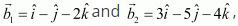respectively .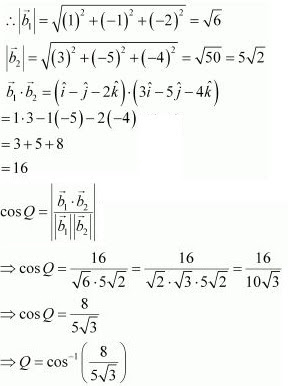11. Find the angle between the following pairs of lines :
(i) (x - 2)/2 = (y - 1)/5 = (z + 3)/-3 and (x +2)/-1 = (y- 4)/8 = (z - 5)/4
(ii) x/2 = y/2 = z/1 and (x -5)/4 = (y - 2)/1 = (z - 3)/8

Solution

(i) Let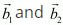be the vectors parallel to the pair of lines, (x-2)/2 = (y-1)/5 = (z+3)/-3 and (x+2)/-1 = (y- 4)/8 = (z-5)/4, respectively.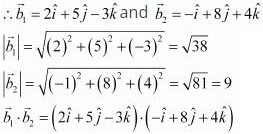= 2(-1) + 5×8 + (-3)×4
= -2 + 40 - 12
= 26
The angle, Q, between the given pair of lines is given by the relation,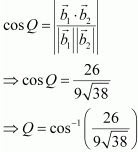(ii)  Let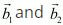be the vectors parallel to the given pair of lines, x/2 = y/2 = z/1 and (x-5)/4 = (y-5)/1 = (z-3)/8, respectively.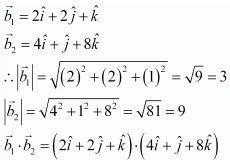= 2×4 + 2×1 + 1×8
= 8 + 2 + 8
= 18

If Q is the angle between the given pair of lines, then cos Q =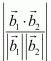⇒ cos Q = 18/(3 × 9) = 2/3
⇒ Q = cos-1 (2/3)

12. Find the values of p so the line (1-x)/3 = (7y-14)/2p = (z-3)/2 and (7-7x)/3p = (y-5)/1 = (6-z)/5 are right angles.

Solution

The given equations can be written in the standard form as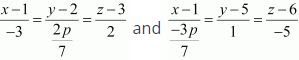The direction ratios of the lines are -3, 2p/7, 2 and -3p/7, 1, -5 respectively.
Two lines with direction ratios, a1, b1, c1 and a2, b2, c2 are perpendicular to each other, if  a1a2 + b1b2 + c1c2 = 0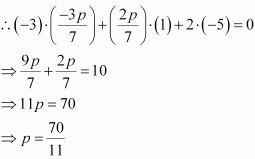Thus, the values of p is 70/11.

13. Show the the lines (x - 5)/7 = (y + 2)/-5 = z/1 and x/1 = y/2 = z/3 are perpendicular to each other.

Solution

The equations of the given lines are (x - 5)/7 =  (y + 2)/-5 = z/1 and x/1 = y/2 = z/3
The direction ratios of the given lines are 7, -5, 1 and 1, 2, 3 respectively.
Two lines with direction ratios, a1 , b1, c1 and a2 , b2, c2 are perpendicular to each other, if  a1a2   + b1b2 + c1c2 = 0
∴ 7×1 + (-5)×2 + 1×3
= 7 - 10 + 3
= 0
Therefore, the given lines are perpendicular to each other.

14. Find the shortest distance between the lines
r⃗ = (i ^- 2j ^+ k ^) + λ (i ^- j ^+ k ^) and
r⃗ = 2i ^- j ^- k + μ (2i ^+ j ^+ 2k ^

Solution

The equations of the given lines are
r⃗ = (i - 2j + k ^) + λ (i - j + k ^
r⃗ = 2i - j - k ^ + μ (2i + j + 2k ^
It is known that the shortest distance between the lines,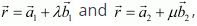is given by,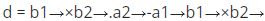Comparing the given equations, we obtain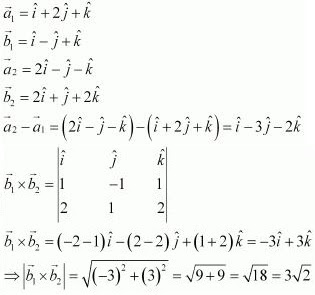Substituting all the values in equation (1), we obtain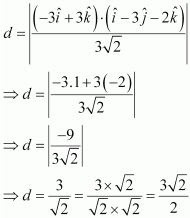Therefore, the shortest distance between the two lines is 3√2/2 units.

15. Find the shortest distance between the lines (x+1)/7 = (y + 1)/-6 = (z + 1)/1 and (x - 3)/1 = (y - 5)/-2 = (z - 7)/1 .

Solution

The given lines are (x+1)/7 = (y + 1)/-6 = (z + 1)/1 and (x - 3)/1 = (y - 5)/-2 = (z - 7)/1 .
It is known that the shortest distance between the two lines,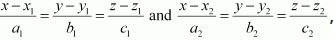is a given by,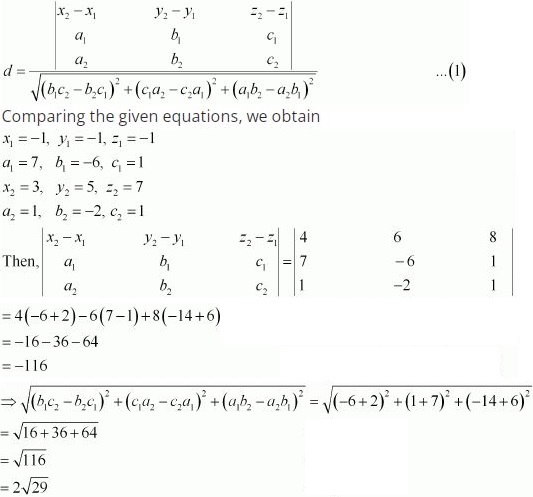Substituting all the values in equation (1), we obtain
d = -116/2√29 = -58/√29 = (-2 × 29)/√29 = -2√29
Since distance is always non-negative, the distance between the given lines is 2√29 units.

16. Find the shortest distance between the lines whose vector equations are
r⃗ = (i ^+ 2j ^+ 3k ^) + λ (i ^- 3j ^+ 2k ^) and
r⃗ = 4i ^+ 5j ^+ 6k ^ + μ (2i ^+ 3j ^+ k ^

Solution

The given lines are  r⃗ = (i + 2j + 3k ^) + λ (i - 3j + 2k ^) and  r⃗ =  4i + 5j + 6k + μ (2i + 3j + k ^
It is known that the shortest distance between the lines,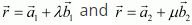is given by,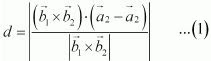Comparing the given equations with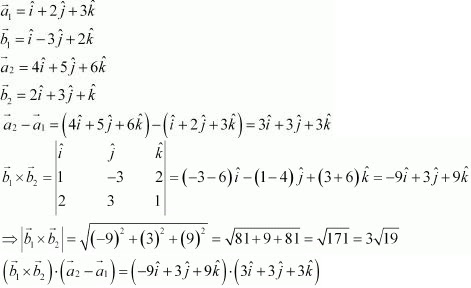= -9×3 + 3×3 + 9×3
= 9
Substituting all the values in equation (1), we obtain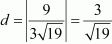Therefore, the shortest distance between the two given lines is 3/√19 units.

17. Find the shortest distance between the lines whose vector equations are
r⃗ = (1 -t)i + (t-2)j + (3-2t) k ^ and
r⃗ = (s + 1)i + (2s- 1)j + (2s+1)k

Solution

The given lines are
r⃗ = (1 -t)i + (t - 2)j + (3 - 2t) k
⇒   r⃗ = (i - 2j + 3k ^) + t(-i + j - 2k ^...(1)
r⃗ =  (s + 1)i + (2s - 1)j + (2s + 1)k
⇒   r⃗ = (i - j - k ^) + s(i +2j - 2k ^...(2)
It is known that the shortest distance between the lines,is given by,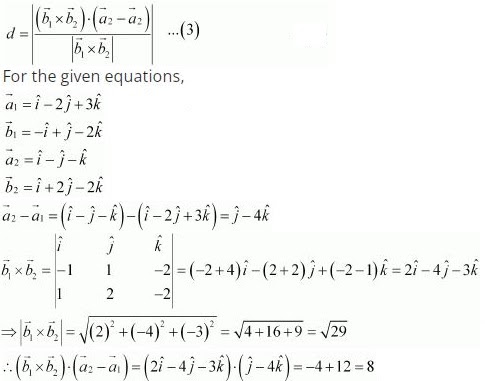Substituting all the values in equation (3), we obtain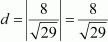Therefore, the shortest distance between the line is 8/√29 units.